【1】什么是单峰区间？什么是单峰函数？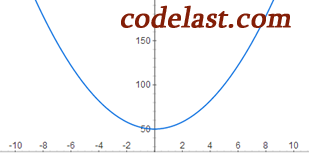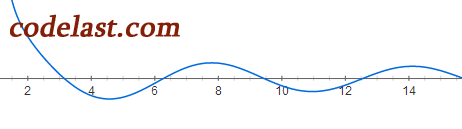$[a,b]$$R$ 的子集，存在 ${\alpha ^*} \in [a,b]$ ，使得 $f(\alpha )$$[a,{\alpha ^*}]$ 上严格单调减，在 $[{\alpha ^*},b]$ 上严格单调增，则称 $[a,b]$$f(\alpha )$ 的单峰区间， $f(\alpha )$$[a,b]$ 上的单峰函数。

【2】“划界”是如何实现的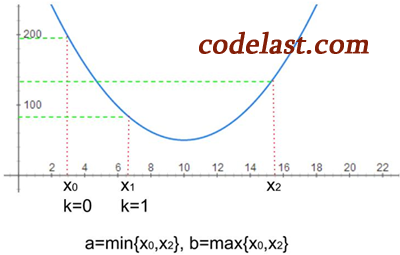${x_1} = {x_0} + h$
${x_2} = {x_1} + t \times h$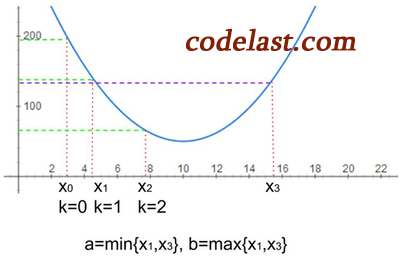${x_1} = {x_0} + h$
${x_2} = {x_1} + t \times h$ （加大步长）
${x_3} = {x_2} + t \times t \times h$ （继续加大步长）

①是向右搜索，如果我们运气更差一些，一开始就是个错误（应该向左搜索），怎么办？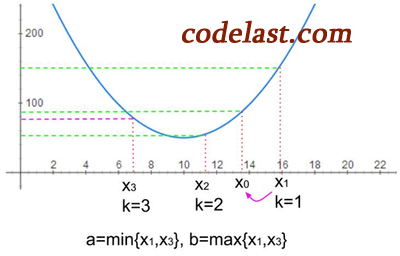${x_1} = {x_0} + h$ （发现函数值不降反升）
$h' = - h$ （步长设为负值，向左搜索）
${x_1} = {x_0}$ （重置 ${x_1}$ 点）
${x_2} = {x_1} + h'$
${x_3} = {x_2} + t \times h'$ （加大步长，函数值回升，停止搜索）

【3】加快划界的速度：逆抛物内插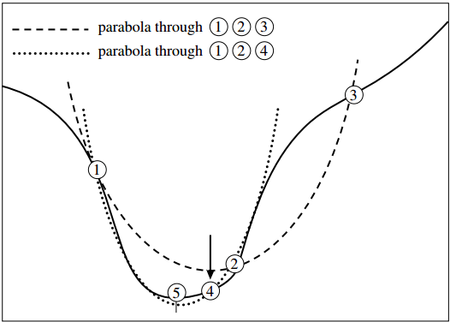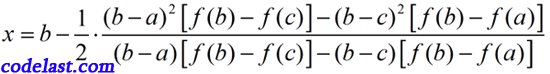[原创]一维搜索中的划界(Bracket)算法
Tagged on:

### One thought on “[原创]一维搜索中的划界(Bracket)算法”

• 2015 年 08 月 18 日 at 22:20
Permalink

那个逆抛物内插是怎么加快速度找到三个点的呢？

Reply
• 2015 年 05 月 22 日 at 14:42
Permalink

楼主写的一系列优化算法非常通俗，调理分明！前段时间一直访问不了楼主的网站，这几天又能访问了！如果能整理成pdf文档那就更好了！

Reply